Project 2: pb-lite: Boundary Detection

Abstract

In this project, I develop an edge detector which utilized information from texture, brightness and color of images by applying a bank of filters to images. After combining the result from a Canny detector, we get quite well results (shown below) on the test set, which is a subset of the Berkeley Segmentation Data Set 500 (BSDS500).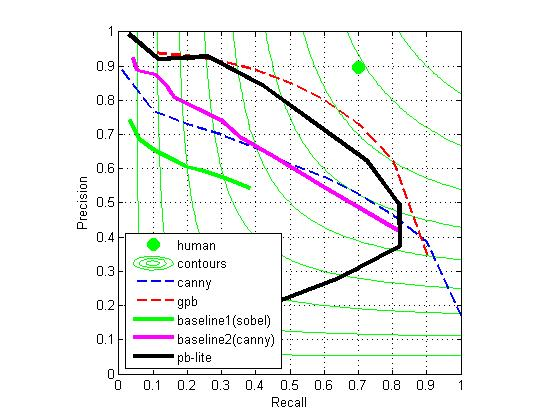In the following part of this report, I will introduce the methods applied in my algorithm to detect the edges. Results of testing on the images in folder "testset" will be given to show the difference of whether some method described in a section is applied. Comparing results from different sections is meaningless.

Filter Bank

I used a set of filter bank with 16 orientated of 3 scaled odd-symmetric Gaussian derivative filters and a center surrounded (difference of Gaussians) filter as shown below.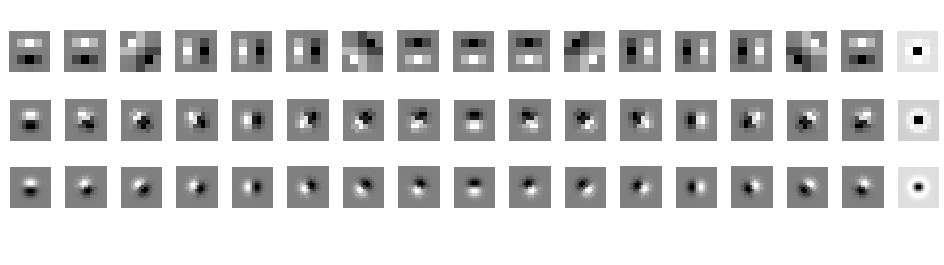The result is very good: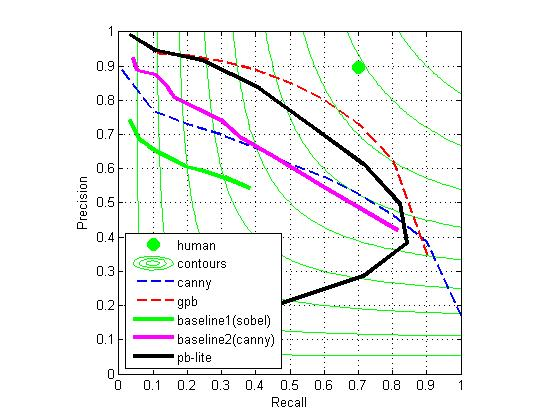I also tried 16 orientated of 3 scaled odd-symmetric Gaussian derivative filters, 16 orientated of 3 scaled even-symmetric Gaussian derivative filters and a center surrounded filter (all other conditions the same), which is suggested in the paper Arbelaez, Maire, Fowlkes, and Malik. TPAMI 2011 (pdf):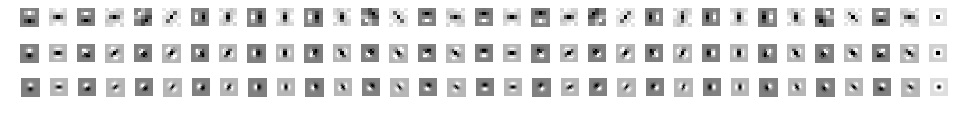the result is not as good as prvious one: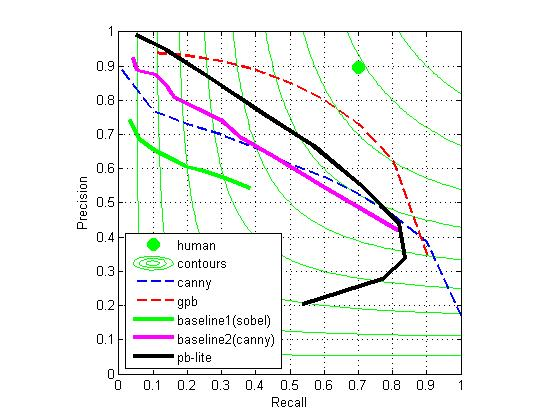When I tried with only 16 orientated of 3 scaled even-symmetric Gaussian derivative filters and a center surrounded filter (with all other parameters same):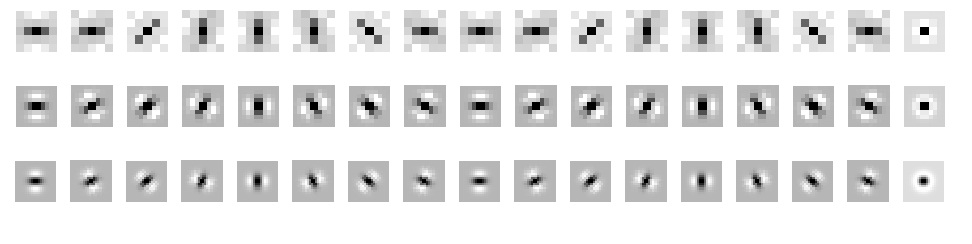The result is similar: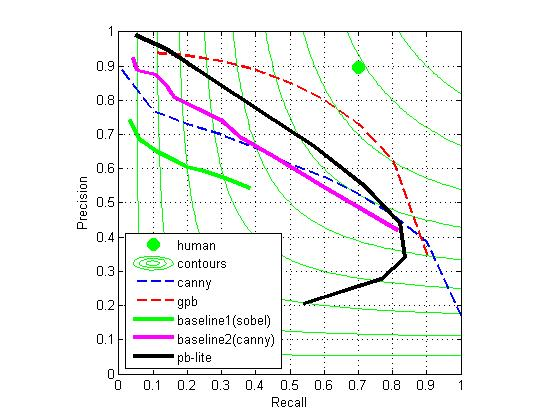I think the reason may be that in a natural image, the edge is usually not in even-symmetric Gaussian pattern. Therefore, the result of only using even-symmetric Gaussian is worse than only using odd-symmetric Gaussian. The reason that the combination doesn't work better than the only even-symmetric Gaussian version may need more exploration, but this is not important in our case, because we only need the best one.

I used 8 pairs of half-disc masks with different orientation in 3 scales. They are binary images of Gaussian Derivative filters. These masks are used for computing chi-square difference between response of the image under two half-discs.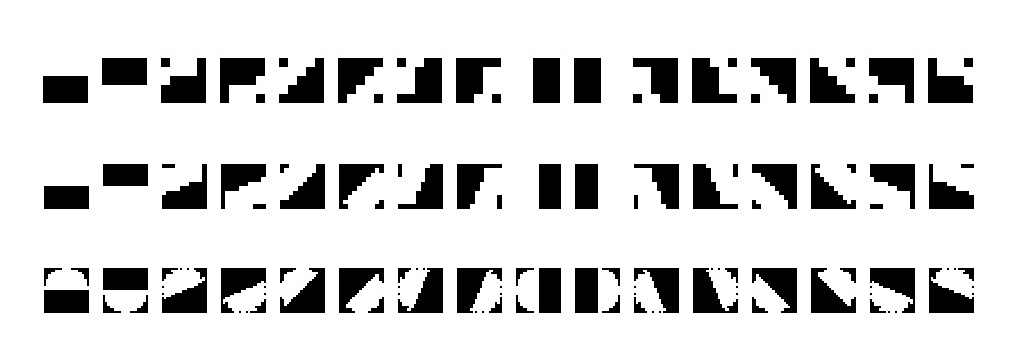Channels

We apply the filter bank and half disk in the four channels, L(brightness),a,b (these two channels are from Lab color space to represent color) and texton map.

Texton map channel

This channel is obtained by first applying the filter bank to the image, and then cluster the vectors of responses of different filters across images.

Lab channels

The reason we use Lab color space to represent brightness and color channels instead of RGB color space is because the difference in Lab color space reflects the perception difference for human beings(according to wikipedia). Therefore, the edge found in Lab space is more likely to be an edge recognized by human beings.

After replacing the brightness channel with Lab channel, obviously, the result of our edge detector improves. The reason behind might be that as we have more information, we can make a wiser decision.

Only brightness and texton channel: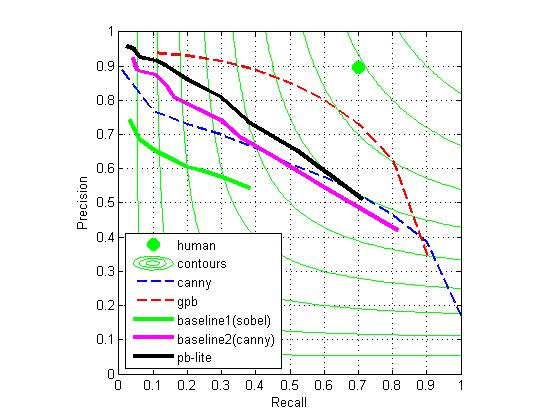Lab and texton channels:Get pb for each channel

As we apply half-disc pairs with 8 orientations and 3 scales to each channel, we get 24 responses. In order to get the final response, we need to combine all those 24 responses together. We choose the max value of all responses instead of the mean value. Since the response reflects the likelyhood of an edge at that orientation, we should choose the most possible orientation of the edge instead of the average orientation. The result also support our reasoning.

Keep all the other edge detecting algorithm and parameters the same, if we use mean value, we got the following pb result:If we use max value:We see the latter result is better than the former result in all sense.

Combine results from each channel

The problem left now is how to combine the response from all channels. A simple way is to take the mean value at each location of all channels, and the result is not bad.However, as in the Berkeley Segmentation Data Set 500 (BSDS500), we already have the ground truth, we can utilize them to make our result better.

For each pixel, we have responses from Lab channels, lg,ag,bg, we have response from texton map, tg, and we also have response from canny detector, cg. We want to find the probability of whether a pixel is an edge, pb, given its lg, ag, bg,tg and cg. Assume pb is a linear combination of lg, ag, bg, tg and cg, then we have

pb=a1*lg+a2*ag+a3*bg+a4*tg+a5*cg

As in the ground thruth, we already have the pb for all pixels, we can choose a set as training set. We can get a vector p which includes all the pb of pixels in the training set. Then we can use our edge detecting algorithm to find the corresponding lg,ag,bg,tg and cg, and save them as rows into a matrix M. Now the problem becomes to find the a=[a1 a2 a3 a4 a5]',such that ||p-M*a|| is minimized.

This is a typical least square problem, and in matlab command notation, we can solve a as

a= inv(M'*M)*(M')*p

Optimally, if we use a in to the equation

pb=a1*lg+a2*ag+a3*bg+a4*tg+a5*cg

we will get the best result. However, to evaluate pb given lg, ag, bg, tg and cg for natrual images is much more complex than a linear combination problem, and apply a directly will have a poor result. For example, when we use the images "35049.jpg" as training set, we get a=[-0.0091,0.0186,0.0299,0.0062,0.0031]. The result of testing on the 10 images in folder "testset" is very poor shown below.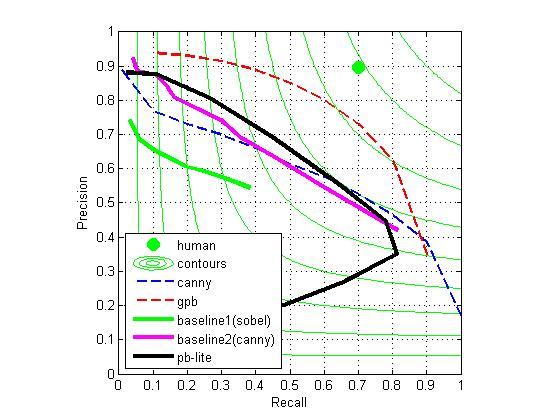however, if we treat a as a hint of the responses from which channel are more important and that from which channel are less important, and assign a1, a2, a3, a4, a5 an appropriate value (as we will normalize pb at last, so only the ratio of a1 a2 a3 a4 a5 matters) according to that, we can get better results than just set all of them 1(which is what we do when we average responses from all channels).

According to the a=[-0.0091,0.0186,0.0299,0.0062,0.0031] we got, we know that the importance is tg>ag>bg>cg>lg, so we set [a1,a2,a3,a4,a5]=[0.6,1.2,1.4,1,0.8], we get a very good result which we showed at first:Shortcomings

As the method we use to find a is very simple, the a we found depends seriously on the training data (large bias). A better way would be to use some machine learning methods, such as SVM, to learn the value of a.

However, as it's not easy to implement(or find) an appropriate machine learning method in such a short period, we are just trying to find some parameters better than averaging the responses from all channels.